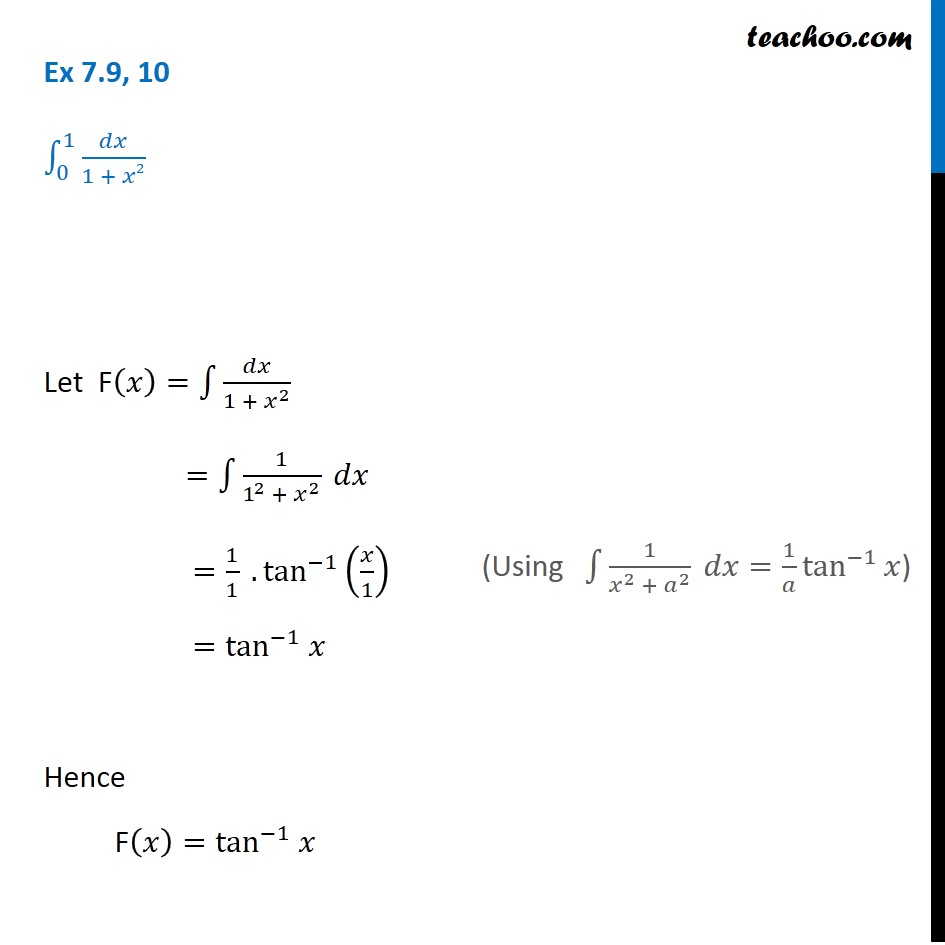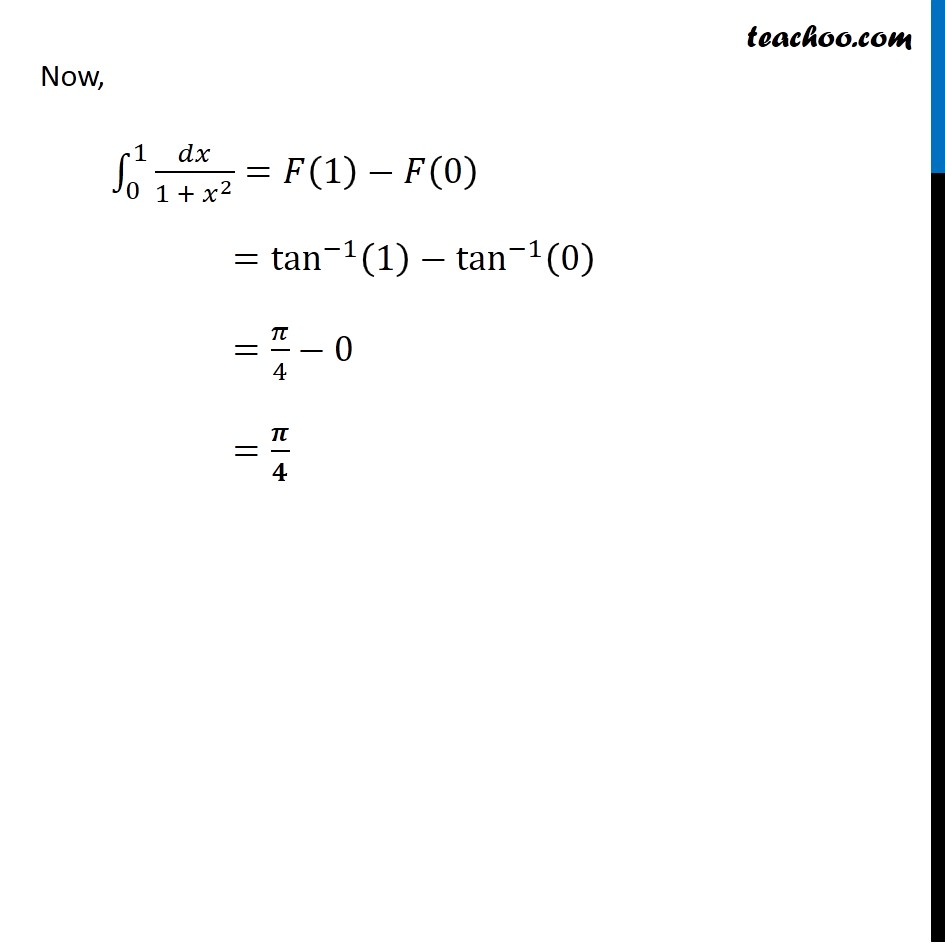Integration Full Chapter Explained - Integration Class 12 - Everything you need1. Chapter 7 Class 12 Integrals
2. Concept wise
3. Definite Integration - By Formulae

Transcript

Ex 7.9, 10 ∫_0^1▒𝑑𝑥/(1 + 𝑥2) Let F(𝑥)=∫1▒𝑑𝑥/(1 + 𝑥^2 ) =∫1▒1/(1^2 + 𝑥^2 ) 𝑑𝑥 =1/1 .tan^(−1)⁡(𝑥/1) =tan^(−1) 𝑥 Hence F(𝑥)=tan^(−1) 𝑥 (Using ∫1▒1/(𝑥^2 + 𝑎^2 ) 𝑑𝑥=1/𝑎 tan^(−1)⁡𝑥) Now, ∫_0^1▒〖𝑑𝑥/(1 + 𝑥^2 )=𝐹(1)−𝐹(0) 〗 =tan^(−1)⁡〖(1)−tan^(−1)⁡(0) 〗 =𝜋/4−0 =𝝅/𝟒

Definite Integration - By Formulae

About the AuthorDavneet Singh
Davneet Singh is a graduate from Indian Institute of Technology, Kanpur. He has been teaching from the past 9 years. He provides courses for Maths and Science at Teachoo.